Topics: AI | Data Science | Data Visualization | Deep Learning | Machine Learning | NLP | Python | R | Statistics

KDnuggets Home » News » 2020 » Jun » Tutorials, Overviews » The 8 Basic Statistics Concepts for Data Science ( 20:n26 )

# The 8 Basic Statistics Concepts for Data Science

Understanding the fundamentals of statistics is a core capability for becoming a Data Scientist. Review these essential ideas that will be pervasive in your work and raise your expertise in the field.

By Shirley Chen, MSBA in ASU | Data Analyst.Statistics is a form of mathematical analysis that uses quantified models and representations for a given set of experimental data or real-life studies. The main advantage of statistics is that information is presented in an easy way. Recently, I reviewed all the statistics materials and organized the 8 basic statistics concepts for becoming a data scientist!

• Understand the Type of Analytics
• Probability
• Central Tendency
• Variability
• Relationship Between Variables
• Probability Distribution
• Hypothesis Testing and Statistical Significance
• Regression

### Understand the Type of Analytics

Descriptive Analytics tells us what happened in the past and helps a business understand how it is performing by providing context to help stakeholders interpret information.

Diagnostic Analytics takes descriptive data a step further and helps you understand why something happened in the past.

Predictive Analytics predicts what is most likely to happen in the future and provides companies with actionable insights based on the information.

Prescriptive Analytics provides recommendations regarding actions that will take advantage of the predictions and guide the possible actions toward a solution.

### Probability

Probability is the measure of the likelihood that an event will occur in a Random Experiment.

Complement: P(A) + P(A’) = 1

Intersection: P(A∩B) = P(A)P(B)

Union: P(A∪B) = P(A) + P(B) − P(A∩B)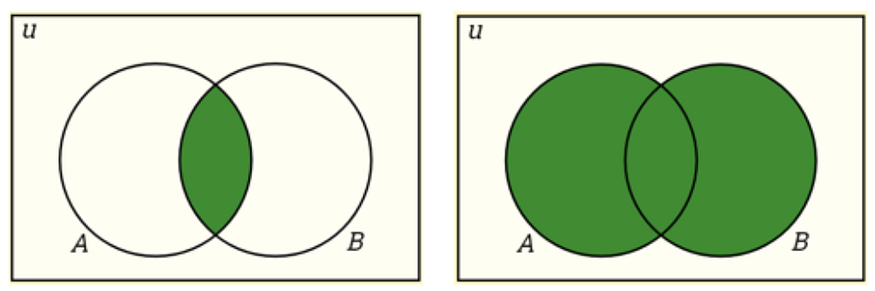Intersection and Union.

Conditional Probability: P(A|B) is a measure of the probability of one event occurring with some relationship to one or more other events. P(A|B)=P(A∩B)/P(B), when P(B)>0.

Independent Events: Two events are independent if the occurrence of one does not affect the probability of occurrence of the other. P(A∩B)=P(A)P(B) where P(A) != 0 and P(B) != 0 , P(A|B)=P(A), P(B|A)=P(B)

Mutually Exclusive Events: Two events are mutually exclusive if they cannot both occur at the same time. P(A∩B)=0 and P(A∪B)=P(A)+P(B).

Bayes’ Theorem describes the probability of an event based on prior knowledge of conditions that might be related to the event.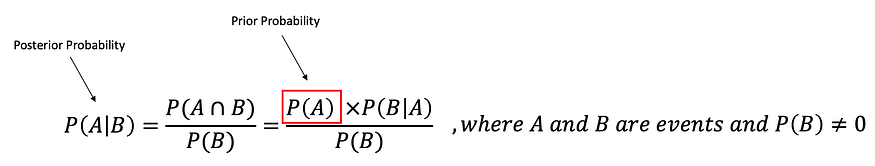Bayes’ Theorem.

### Central Tendency

Mean: The average of the dataset.

Median: The middle value of an ordered dataset.

Mode: The most frequent value in the dataset. If the data have multiple values that occurred the most frequently, we have a multimodal distribution.

Skewness: A measure of symmetry.

Kurtosis: A measure of whether the data are heavy-tailed or light-tailed relative to a normal distribution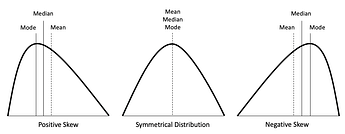Skewness.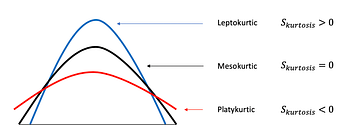Kurtosis.

### Variability

Range: The difference between the highest and lowest value in the dataset.

Percentiles, Quartiles and Interquartile Range (IQR)

• Percentiles — A measure that indicates the value below which a given percentage of observations in a group of observations falls.
• Quantiles— Values that divide the number of data points into four more or less equal parts, or quarters.
• Interquartile Range (IQR)— A measure of statistical dispersion and variability based on dividing a data set into quartiles. IQR = Q3 − Q1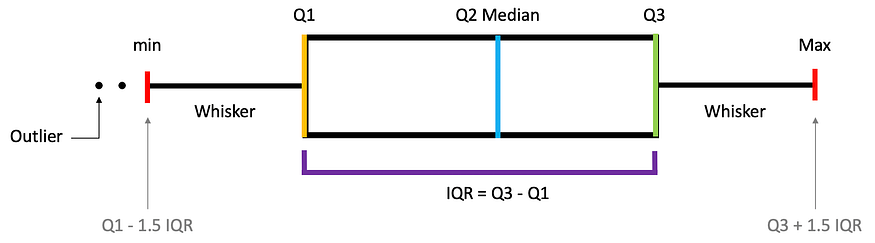Percentiles, Quartiles and Interquartile Range (IQR).

Variance: The average squared difference of the values from the mean to measure how spread out a set of data is relative to mean.

Standard Deviation: The standard difference between each data point and the mean and the square root of variance.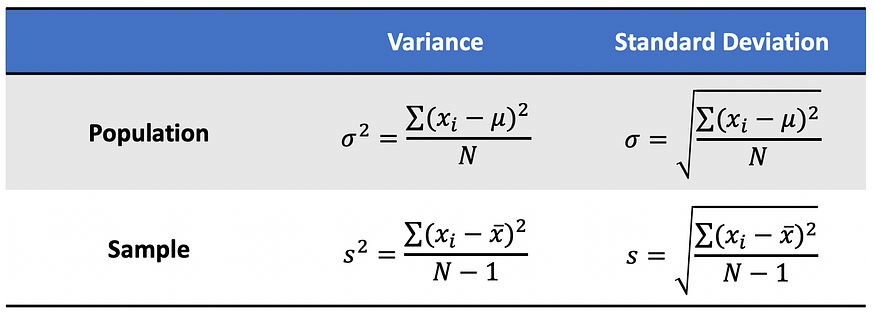Population and Sample Variance and Standard Deviation.

Standard Error (SE): An estimate of the standard deviation of the sampling distribution.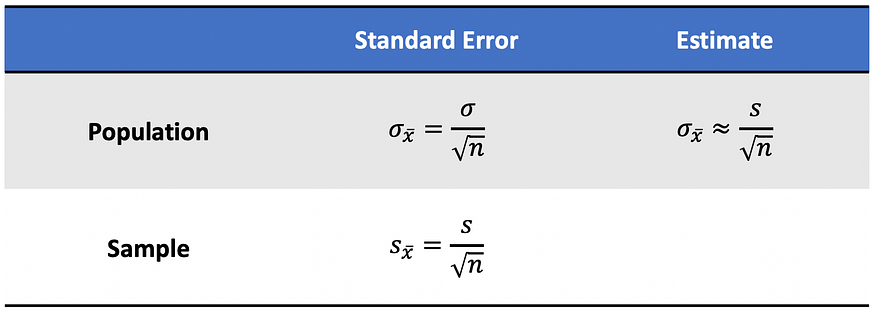Population and Sample Standard Error.

### Relationship Between Variables

Causality: Relationship between two events where one event is affected by the other.

Covariance: A quantitative measure of the joint variability between two or more variables.

Correlation: Measure the relationship between two variables and ranges from -1 to 1, the normalized version of covariance.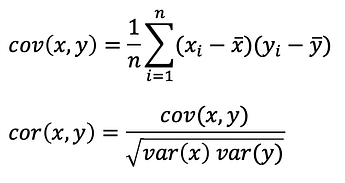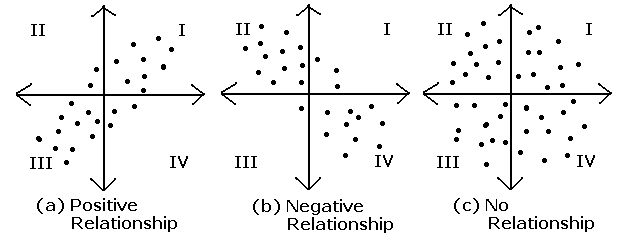Covariance and Correlation.

### Probability Distributions

Probability Distribution Functions

Probability Mass Function (PMF): A function that gives the probability that a discrete random variable is exactly equal to some value.

Probability Density Function (PDF): A function for continuous data where the value at any given sample can be interpreted as providing a relative likelihood that the value of the random variable would equal that sample.

Cumulative Density Function (CDF): A function that gives the probability that a random variable is less than or equal to a certain value.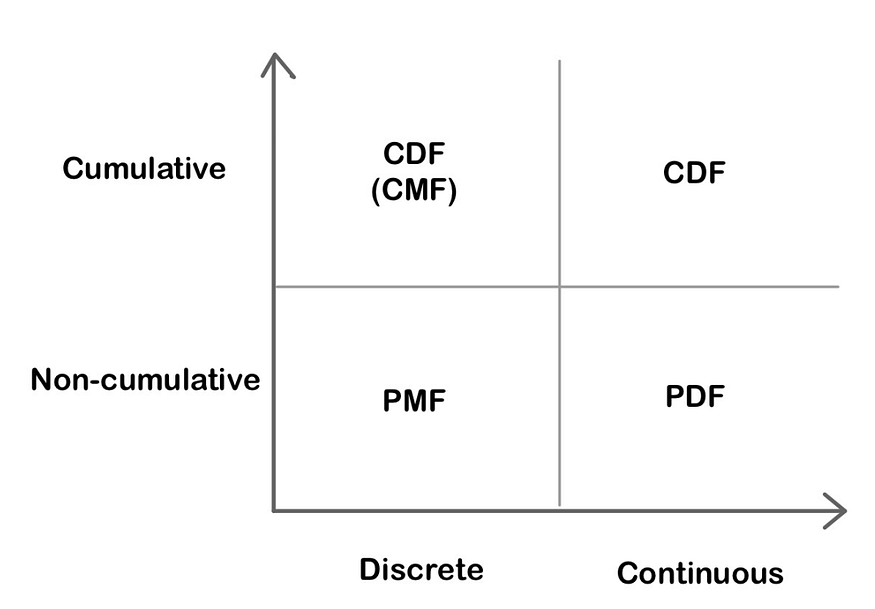Comparison between PMF, PDF, and CDF.

Continuous Probability Distribution

Uniform Distribution: Also called a rectangular distribution, is a probability distribution where all outcomes are equally likely.

Normal/Gaussian Distribution: The curve of the distribution is bell-shaped and symmetrical and is related to the Central Limit Theorem that the sampling distribution of the sample means approaches a normal distribution as the sample size gets larger.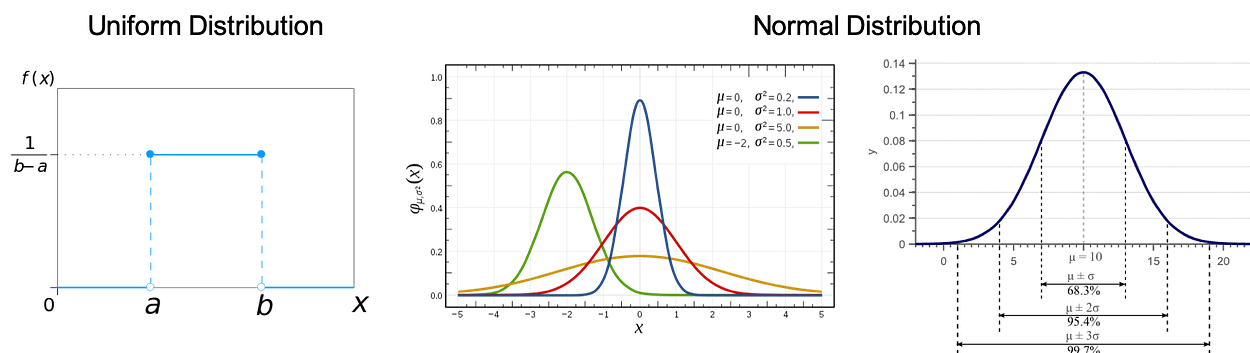Exponential Distribution: A probability distribution of the time between the events in a Poisson point process.

Chi-Square Distribution: The distribution of the sum of squared standard normal deviates.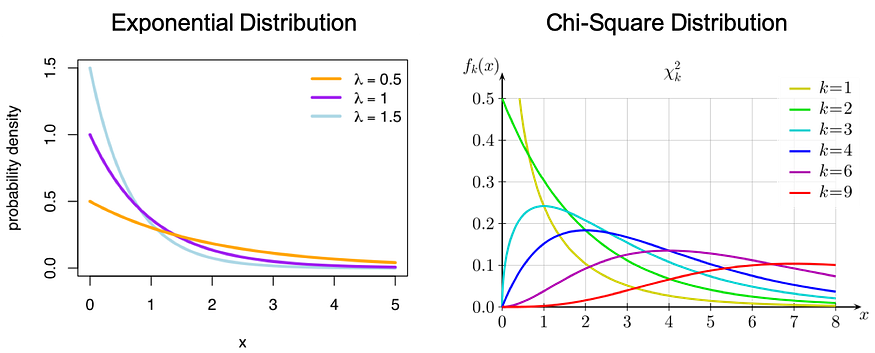Discrete Probability Distribution

Bernoulli Distribution: The distribution of a random variable which takes a single trial and only 2 possible outcomes, namely 1(success) with probability p, and 0(failure) with probability (1-p).

Binomial Distribution: The distribution of the number of successes in a sequence of n independent experiments, and each with only 2 possible outcomes, namely 1(success) with probability p, and 0(failure) with probability (1-p).

Poisson Distribution: The distribution that expresses the probability of a given number of events k occurring in a fixed interval of time if these events occur with a known constant average rate λ and independently of the time.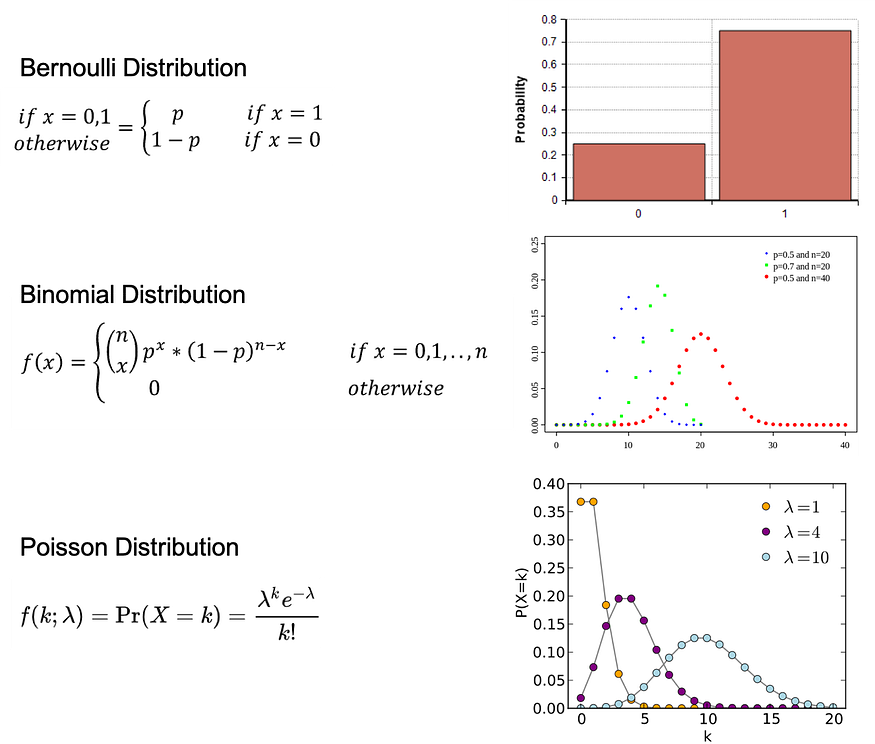### Hypothesis Testing and Statistical Significance

Null and Alternative Hypothesis

Null Hypothesis: A general statement that there is no relationship between two measured phenomena or no association among groups. Alternative Hypothesis: Be contrary to the null hypothesis.

In statistical hypothesis testing, a type I error is the rejection of a true null hypothesis, while a type II error is the non-rejection of a false null hypothesis.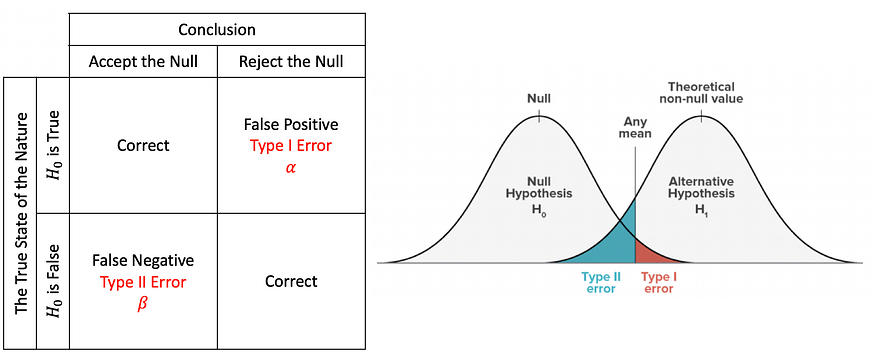Interpretation

P-value: The probability of the test statistic being at least as extreme as the one observed given that the null hypothesis is true. When p-value > α, we fail to reject the null hypothesis, while p-value ≤ α, we reject the null hypothesis, and we can conclude that we have a significant result.

Critical Value: A point on the scale of the test statistic beyond which we reject the null hypothesis and is derived from the level of significance α of the test. It depends upon a test statistic, which is specific to the type of test, and the significance level, α, which defines the sensitivity of the test.

Significance Level and Rejection Region: The rejection region is actually dependent on the significance level. The significance level is denoted by α and is the probability of rejecting the null hypothesis if it is true.

Z-Test

Z-test is any statistical test for which the distribution of the test statistic under the null hypothesis can be approximated by a normal distribution and tests the mean of a distribution in which we already know the population variance. Therefore, many statistical tests can be conveniently performed as approximate Z-tests if the sample size is large or the population variance is known.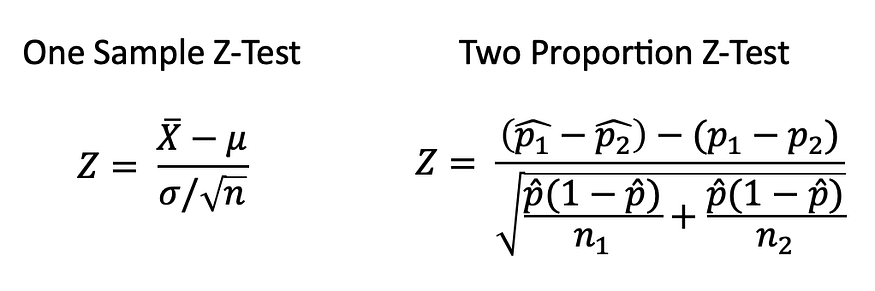T-Test

A T-test is the statistical test if the population variance is unknown, and the sample size is not large (n < 30).

Paired sample means that we collect data twice from the same group, person, item, or thing. Independent sample implies that the two samples must have come from two completely different populations.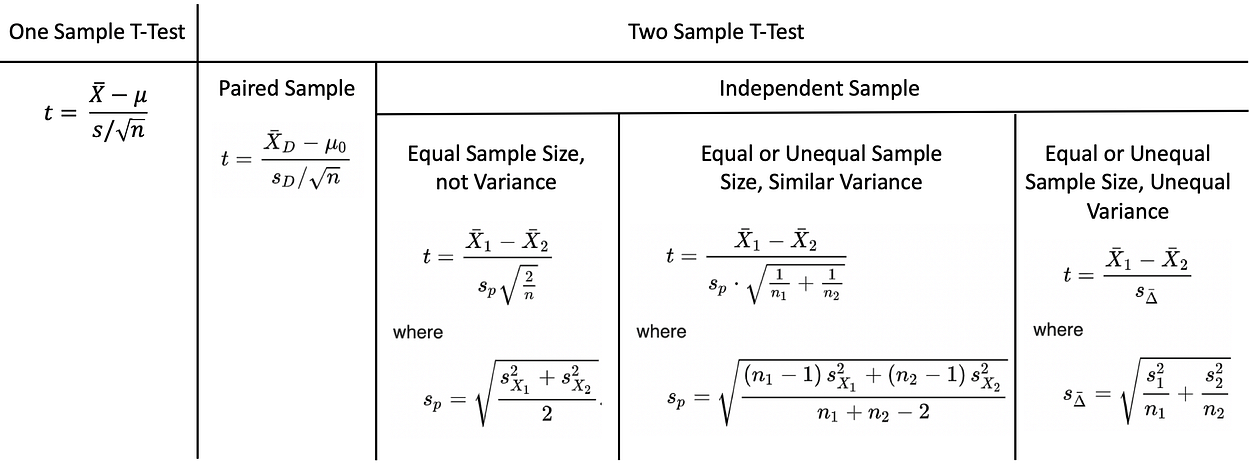ANOVA (Analysis of Variance)

ANOVA is the way to find out if experimental results are significant. One-way ANOVA compares two means from two independent groups using only one independent variable. Two-way ANOVA is the extension of one-way ANOVA using two independent variables to calculate the main effect and interaction effect.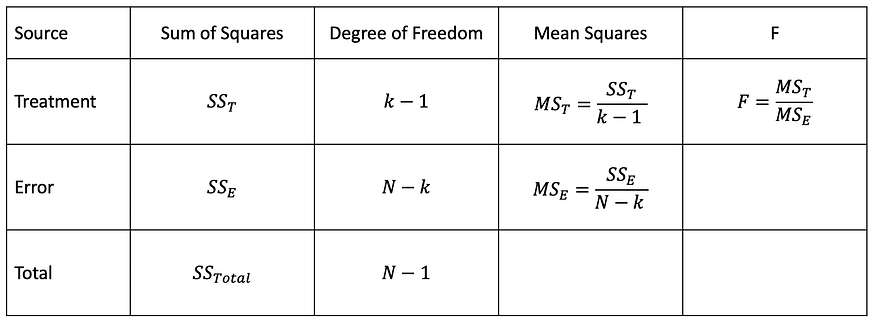ANOVA Table.

Chi-Square Test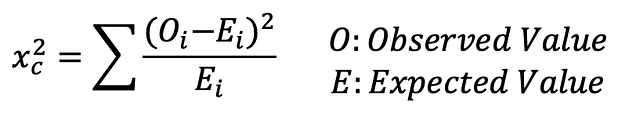Chi-Square Test Formula.

Chi-Square Test checks whether or not a model follows approximately normality when we have s discrete set of data points. Goodness of Fit Test determines if a sample matches the population fit one categorical variable to a distribution. Chi-Square Test for Independence compares two sets of data to see if there is a relationship.

### Regression

Linear Regression

Assumptions of Linear Regression

• Linear Relationship
• Multivariate Normality
• No or Little Multicollinearity
• No or Little Autocorrelation
• Homoscedasticity

Linear Regression is a linear approach to modeling the relationship between a dependent variable and one independent variable. An independent variable is a variable that is controlled in a scientific experiment to test the effects on the dependent variable. A dependent variable is a variable being measured in a scientific experiment.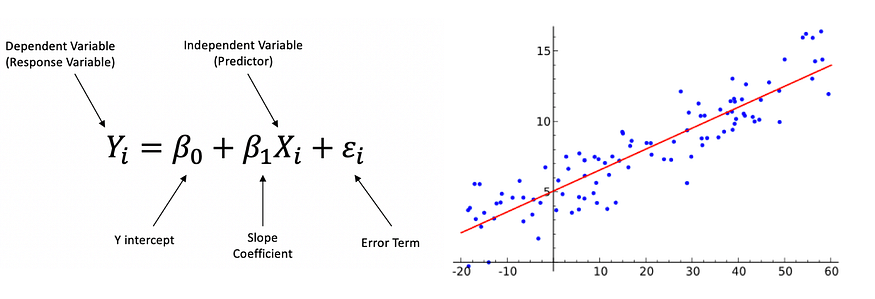Linear Regression Formula.

Multiple Linear Regression is a linear approach to modeling the relationship between a dependent variable and two or more independent variables.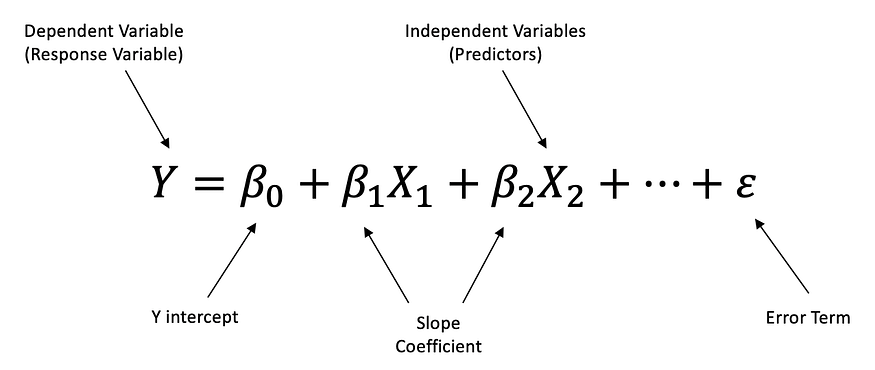Multiple Linear Regression Formula.

Steps for Running the Linear Regression

Step 1: Understand the model description, causality, and directionality

Step 2: Check the data, categorical data, missing data, and outliers

• Outlier is a data point that differs significantly from other observations. We can use the standard deviation method and interquartile range (IQR) method.
• Dummy variable takes only the value 0 or 1 to indicate the effect for categorical variables.

Step 3: Simple Analysis — Check the effect comparing between dependent variable to independent variable and independent variable to independent variable

• Use scatter plots to check the correlation
• Multicollinearity occurs when more than two independent variables are highly correlated. We can use Variance Inflation Factor (VIF) to measure if VIF > 5 there is highly correlated and if VIF > 10, then there is certainly multicollinearity among the variables.
• Interaction Term implies a change in the slope from one value to another value.

Step 4: Multiple Linear Regression — Check the model and the correct variables

Step 5: Residual Analysis

• Check normal distribution and normality for the residuals.
• Homoscedasticity describes a situation in which the error term is the same across all values of the independent variables and means that the residuals are equal across the regression line.

Step 6: Interpretation of Regression Output

• R-Squared is a statistical measure of fit that indicates how much variation of a dependent variable is explained by the independent variables. Higher R-Squared value represents smaller differences between the observed data and fitted values.
• P-value
• Regression Equation

Original. Reposted with permission.

Bio: Shirley Chen is a Business Intelligence Analyst at U-Haul and recent graduate with a Master's Degree in MS-Business Analytics from ASU.

Related:

Top Stories Past 30 Days
Most Popular
Most SharedGet KDnuggets, a leading newsletter on AI, Data Science, and Machine Learning